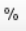# How To Show Percentage Signs On Tables And Plots

Where the Statistic being shown on a table or a chart is naturally a percentage, you can press theto display or hide the percentage sign.

In some situations, such as when computing Net Promoter Score the results of a calculate may be interpretable as percentages, but this button will not be available, as the statistic in which the outputs are stored, typically the Average, are not percentages.

A workaround is to:

1. Place a statistic on the table that contains % in its name. The example below assumes that this statistic is % Column Share; if you use a different statistic, make sure that you modify the code below.
2. Insert some Custom Rule to transfer the results from one statistic to another statistic that contains percentages. An example, which inserts the Average data into % Column Share is shown below. This code also renames the statistic as Net Promoter Score.
3. Remove the original statistic from the table.
4. If necessary, press.
var n_rows = table.numberRows;
var n_columns = table.numberColumns;
var averages = table.get("Average");
var percents = table.get("% Column Share");
for (var r = 0; r < n_rows; r++)
for (var c = 0; c < n_columns; c++)
percents[r][c] = averages[r][c];
table.set("% Column Share", percents);
form.setTranslation("% Total Share","Net Promoter Score");